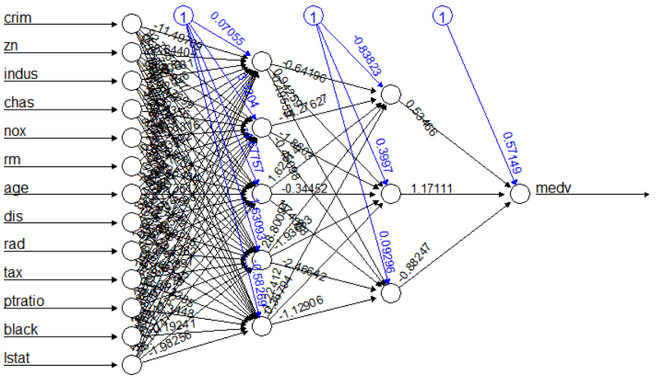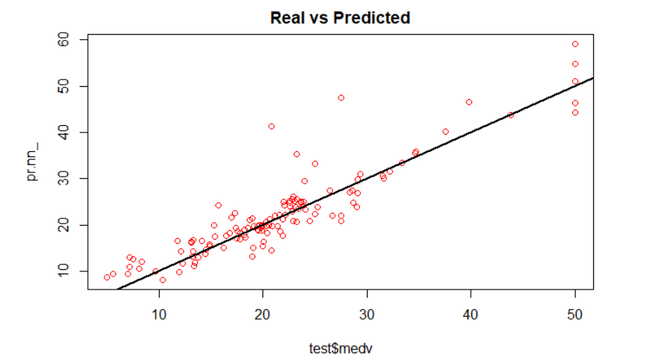Related Articles
How Neural Networks are used for Regression in R Programming?
• Last Updated : 10 Nov, 2020

Neural networks consist of simple input/output units called neurons (inspired by neurons of the human brain). These input/output units are interconnected and each connection has a weight associated with it. Neural networks are flexible and can be used for both classification and regression. In this article, we will see how neural networks can be applied to regression problems.

Regression helps in establishing a relationship between a dependent variable and one or more independent variables. Regression models work well only when the regression equation is a good fit for the data. Most regression models will not fit the data perfectly. Although neural networks are complex and computationally expensive, they are flexible and can dynamically pick the best type of regression, and if that is not enough, hidden layers can be added to improve prediction.

### Stey by Step Implementation in R

Now let us construct a neural network in R programming which solves a regression problem. We will use the Boston dataset to predict the median value of owner-occupied homes (per 1000 dollars).

Step 1: Load the dataset as follows

## R

 `# Import Required packages ` `set.seed``(500) ` `library``(neuralnet) ` `library``(MASS) ` ` `  `# Boston dataset from MASS ` `data <- Boston`

Step 2: Before feeding the data into a neural network, it is good practice to perform normalization. There is a number of ways to perform normalization. We will use the min-max method and scale the data in the interval [0,1]. The data is then split into training (75%) and testing (25%) set.

## R

 `# Normalize the data ` `maxs <- ``apply``(data, 2, max)  ` `mins <- ``apply``(data, 2, min) ` `scaled <- ``as.data.frame``(``scale``(data, center = mins,  ` `                              ``scale = maxs - mins)) ` ` `  `# Split the data into training and testing set ` `index <- ``sample``(1:``nrow``(data), ``round``(0.75 * ``nrow``(data))) ` `train_ <- scaled[index,] ` `test_ <- scaled[-index,]`

Step 3: Now, we can create a neural network using the neuralnet library. Modify the parameters and calculate the mean squared error (MSE). Use the parameters with the least MSE. We will use two hidden layers having 5 and 3 neurons. The number of neurons should be between the input layer size and the output layer size, usually 2/3 of the input size. However, modifying and testing the neural network, again and again, is the best way to find the parameters that best fit your model. When this neural network is trained, it will perform gradient descent to find coefficients that fit the data until it arrives at the optimal weights (in this case regression coefficients) for the model.

## R

 `# Build Neural Network ` `nn <- ``neuralnet``(medv ~ crim + zn + indus + chas + nox  ` `                ``+ rm + age + dis + rad + tax +  ` `                ``ptratio + black + lstat,  ` `                ``data = train_, hidden = ``c``(5, 3),  ` `                ``linear.output = ``TRUE``) ` ` `  `# Predict on test data ` `pr.nn <- ``compute``(nn, test_[,1:13]) ` ` `  `# Compute mean squared error ` `pr.nn_ <- pr.nn\$net.result * (``max``(data\$medv) - ``min``(data\$medv))  ` `                                              ``+ ``min``(data\$medv) ` `test.r <- (test_\$medv) * (``max``(data\$medv) - ``min``(data\$medv)) +  ` `                                              ``min``(data\$medv) ` `MSE.nn <- ``sum``((test.r - pr.nn_)^2) / ``nrow``(test_) ` ` `  `# Plot the neural network ` `plot``(nn)`

Output:Step 4: We can see in the plot that the black lines show connections between each layer with its weights and the blue lines show the bias added in each step. The neural network is essentially a black box so we cannot say much about the weights.

## R

 `# Plot regression line ` `plot``(test\$medv, pr.nn_, col = ``"red"``,  ` `     ``main = ``'Real vs Predicted'``) ` `abline``(0, 1, lwd = 2)`

Output:We can see that the predictions (red circles) made by the neural network are in general concentrated around the line (a perfect alignment with the line would indicate an MSE of 0 and thus an ideal prediction).My Personal Notes arrow_drop_up
Recommended Articles
Page :